# Order of Operations Worksheets

#### What is the Order of Operations?

Operations usually mean arithmetic ones like add, subtract, multiply, or division. It can also deal with taking power, solving brackets, etc. However, this is a distinct way in which order these operators must be solved. Now, there are different ways in which we perform operations. However, one method that stands out from the rest is the BODMAS method. The BODMAS value means Brackets, Order, Divide, Multiply, Add, and finally Subtract. So, for an expression that consists of all of the above, let’s follow the rules sequentially. 22 + (4 × 3) - 3. The first step is to solve the bracket. In this case, the answer is 12. 22 + 12 - 3. Now, the next step is we’ll solve the power; for which the expression will become: 4 + 12 - 3. The final step is to add the numbers and then subtract three from the answer. 13###### Two Step Problems (Three Factors)

Solve this series of problems that include three factors, subtraction, addition, and multiplication.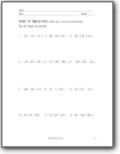###### Three Step Problems (with parentheses)

Solve this series of problems that include four factors, subtraction, addition, multiplication, and parentheses.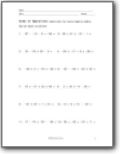###### Three Step Problems (no parentheses - four factors)

All factors are double digits and all operators are on the table for this one.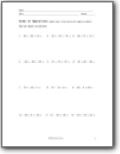###### Two Step Problems (with negative numbers)

The negative numbers may trip up some students.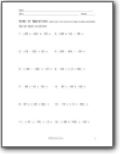###### Mixed Step Problems (with negative numbers and parentheses)

We unleash all the skills in one pack here.###### Mixed Step Problems (single digit-three factors-with negative numbers)

The outcome of the operations are not the point here, it is all about how you arrange you operators.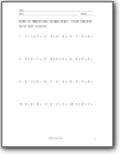###### Two Moves (single digit-three factors-with negative numbers)

These are quick, but the difference is that we include the division operator in here.###### Three Moves (single digit-three factors-with negative numbers)

We throw the whole kitchen sink at you.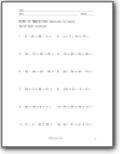###### Operational Moves (single and double digit - four factors)

We just increase the number of factors and start off with no division.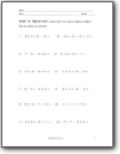###### More Moves (single digit - four factors - negatives)

We just increase the number of factors and start off with no division.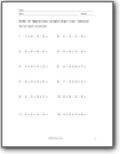###### Four Factors and Simple

As the name says, single digit values with three operators to work with.###### 2 Steps Independent Practice

All problems here require just two steps to solve.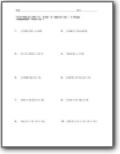###### 3 Steps Independent Practice

You will have to shuffle around a few brackets in here.